# Geometric Sequence Worksheet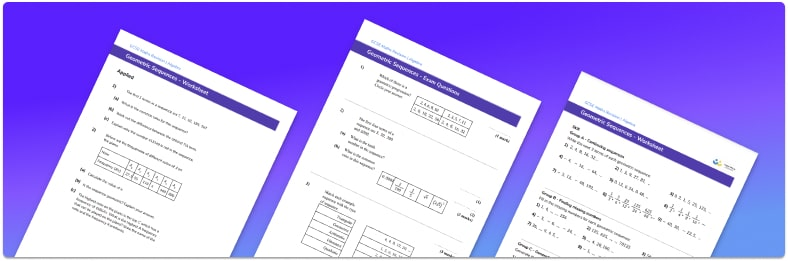• This field is for validation purposes and should be left unchanged.

You can unsubscribe at any time (each email we send will contain an easy way to unsubscribe). To find out more about how we use your data, see our privacy policy.

• Section 1 of the geometric sequence worksheet contains 27 skills-based geometric sequence questions, in 3 groups to support differentiation
• Section 2 contains 3 applied geometric sequence questions with a mix of worded problems and deeper problem solving questions
• Section 3 contains 8 foundation and higher level GCSE exam practice questions
• Answers and a mark scheme for all questions are provided
• Questions follow variation theory with plenty of opportunities for students to work independently at their own level
• All questions created by fully qualified expert secondary maths teachers
• Suitable for GCSE maths revision for AQA, OCR and Edexcel exam boards

### Geometric sequence at a glance

A geometric sequence is a number sequence with a common ratio. The number sequence may have integers, fractions, decimals or a combination.

The common ratio can be found by dividing consecutive terms of the geometric sequence and can be used to find the next term in the sequence by multiplying the previous term by the common ratio. The common ratio can also be used to find other missing terms in the sequence by multiplying or dividing terms by it.

If the number sequence is increasing this means that the common ratio is greater than one. If the number sequence is decreasing this means that the common ratio is less than one.

The first term and the common ratio can be used to write the nth term of a geometric sequence (or position-to-term formula).

Looking forward, students can then progress to additional algebra worksheets, for example a simplifying expressions worksheet or simultaneous equations worksheet.For more teaching and learning support on Algebra our GCSE maths lessons provide step by step support for all GCSE maths concepts.

## Related worksheets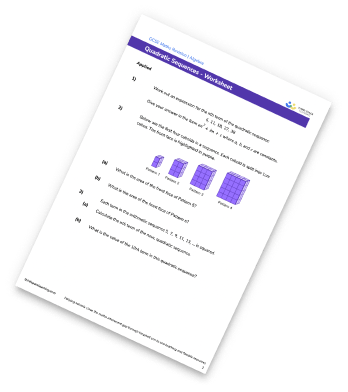Arithmetic Sequence Worksheet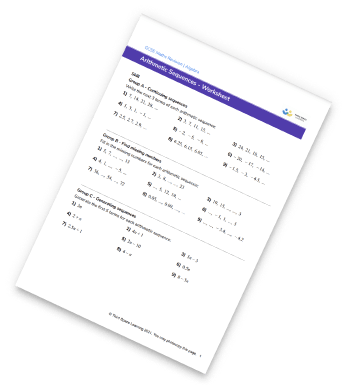Nth Term Worksheet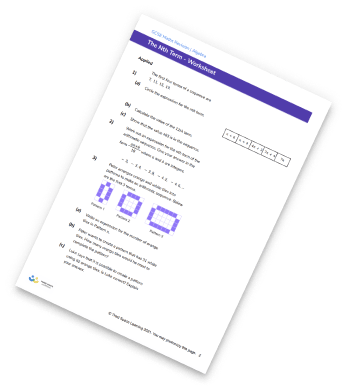Sequences Worksheet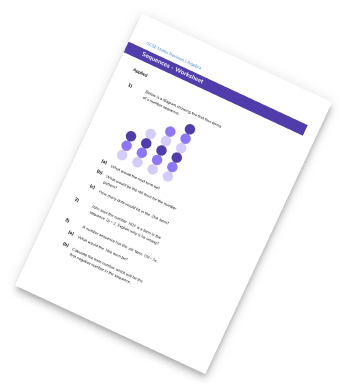## Do you have KS4 students who need more focused attention to succeed at GCSE?There will be students in your class who require individual attention to help them succeed in their maths GCSEs. In a class of 30, it’s not always easy to provide.

Help your students feel confident with exam-style questions and the strategies they’ll need to answer them correctly with our dedicated GCSE maths revision programme.

Lessons are selected to provide support where each student needs it most, and specially-trained GCSE maths tutors adapt the pitch and pace of each lesson. This ensures a personalised revision programme that raises grades and boosts confidence.

Find out more